Question

# Find the vapor vapor compostion of the liquid mixture described below at a temperature of 190...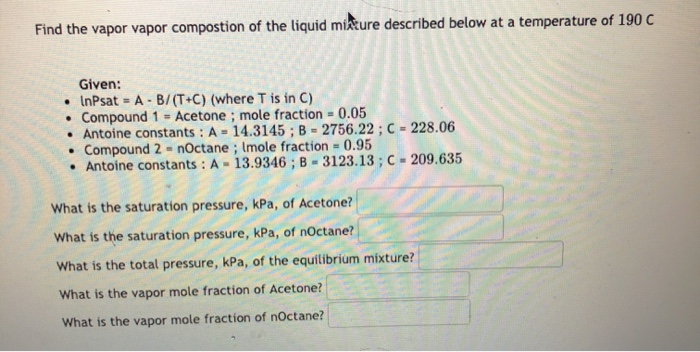Find the vapor vapor compostion of the liquid mixture described below at a temperature of 190 C . Given: • InPsat = A - B/(T+C) (where T is in C) Compound 1 = Acetone; mole fraction = 0.05 • Antoine constants : A = 14.3145 ; B = 2756.22 ; C - 228.06 Compound 2 = nOctane; Imole fraction = 0.95 • Antoine constants: A - 13.9346; B - 3123.13 ; C - 209.635 . What is the saturation pressure, kPa, of Acetone? What is the saturation pressure, kPa, of nOctane? What is the total pressure, kPa, of the equilibrium mixture? What is the vapor mole fraction of Acetone? What is the vapor mole fraction of nOctane?

We need at least 10 more requests to produce the answer.

0 / 10 have requested this problem solution

The more requests, the faster the answer.

All students who have requested the answer will be notified once they are available.

#### Earn Coins

Coins can be redeemed for fabulous gifts.

Similar Homework Help Questions
• ### Find the vapor pressure, kPa, at a temperature of 90 C Given: • Compound = nOctane...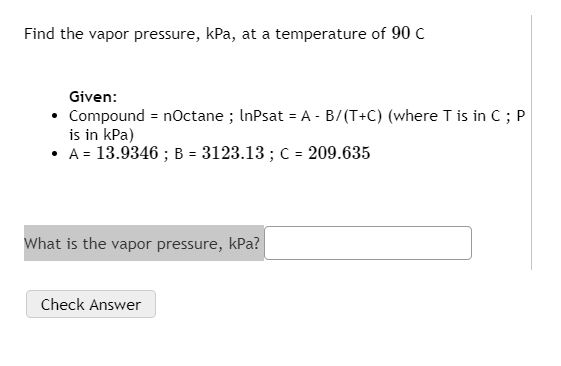Find the vapor pressure, kPa, at a temperature of 90 C Given: • Compound = nOctane ; InPsat = A - B/(T+C) (where T is in C;P is in kPa) • A = 13.9346 ; B = 3123.13 ; C = 209.635 What is the vapor pressure, kPa? Check Answer

• ### ourse Compounds 1 and 2 are in equilibrium at 165 C and 792.16 bar Modified Raoult's...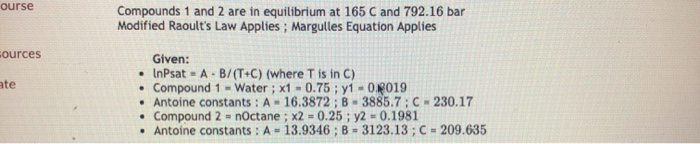ourse Compounds 1 and 2 are in equilibrium at 165 C and 792.16 bar Modified Raoult's Law Applies ; Margulles Equation Applies ources ate Given: • InPsat = A - B/(T+C) (where T is in C) • Compound 1 - Water ; x1 -0.75 ; y1 -0.2019 • Antoine constants : A - 16.3872; B - 3885.7: C - 230.17 Compound 2 = nOctane ; x2 = 0.25 : y2 = 0.1981 . Antoine constants : A - 13.9346; B...

• ### Q3. (7.5 marks) A gas mixture containing 88.0 mol% N2 and 12.0 mol% ethanol vapor, flows...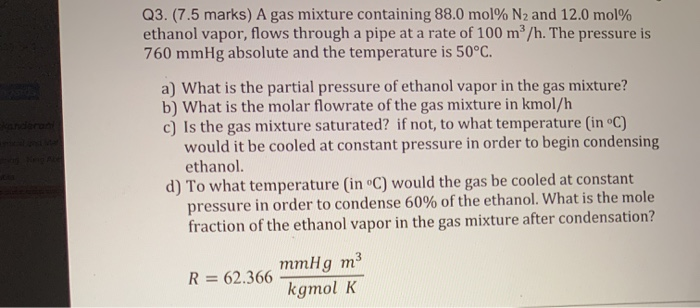Q3. (7.5 marks) A gas mixture containing 88.0 mol% N2 and 12.0 mol% ethanol vapor, flows through a pipe at a rate of 100 m/h. The pressure is 760 mmHg absolute and the temperature is 50°C. a) What is the partial pressure of ethanol vapor in the gas mixture? b) What is the molar flowrate of the gas mixture in kmol/h c) Is the gas mixture saturated? if not, to what temperature (in °C) would it be cooled at constant...

• ### Find the mole fractions in the liquid mixture and in the vapor phase

At 100 ?C the vapor pressures of hexane and octane are 1836 and 354 torr, respectively. A certain liquid mixture of these two compounds has a vapor pressure of 666torr at 100 ?C. Find the mole fractions in the liquid mixture and in the vapor phase. Assume an ideal solution.

• ### the liquid and ya the mole fraction in the vapor.) 8. At 90°C, the vapor pressure...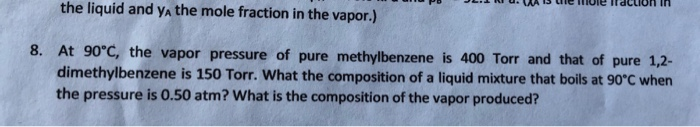the liquid and ya the mole fraction in the vapor.) 8. At 90°C, the vapor pressure of pure methylbenzene is 400 Torr and that of pure 1,2- dimethylbenzene is 150 Torr. What the composition of a liquid mixture that boils at 90°C when the pressure is 0.50 atm? What is the composition of the vapor produced?

• ### 1. As the mole fraction of chloroform approaches 1, the vapor pressure of acetone could be...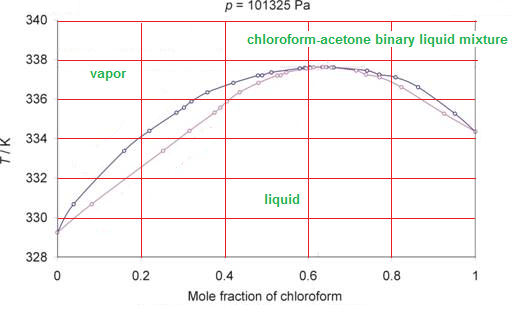1. As the mole fraction of chloroform approaches 1, the vapor pressure of acetone could be calculated using A. Raoult's Law. B. Henry's Law. 2.If a chloroform-acetone mixture with an chloroform mole fraction of 0.62 is subjected to fractional distillation, what is the composition of the distillate? A. pure chloroform B. pure azeotrope C. pure acetone 3. Do chloroform and acetone form an ideal solution? A. no B. cannot be determined from the information given C. yes 4. If a...

• ### Diagram 1 Liquid-Vapor Phase Diagram for a Binary Mixture of A and B 90 80 Temp...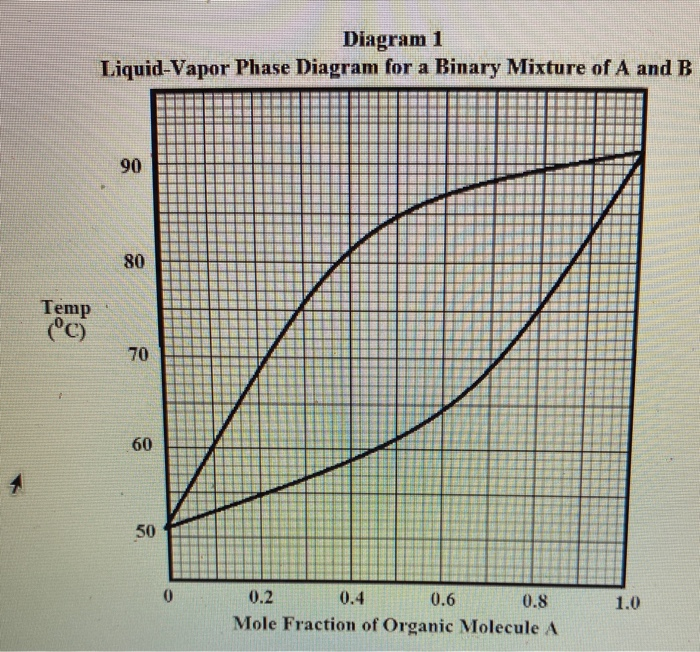Diagram 1 Liquid-Vapor Phase Diagram for a Binary Mixture of A and B 90 80 Temp (°C) 70 60 50 0.6 1.0 0.2 0.4 0.8 Mole Fraction of Organic Molecule A Refer to the Diagram 1 The liquid-vapor phase diagram for mixtures of Organic A (molar mass: 96.2 g/mole) and Organic B (molar mass = 70.21 g/mole). From the diagram, the boiling point of pure Organic A is: REPORT YOUR ANSWER TO 2 DIGITS ACCURACY DO NOT PUT UNITS IN...

• ### Part A Two liquids, labeled A and B, form an ideal mixture with total vapor pressure...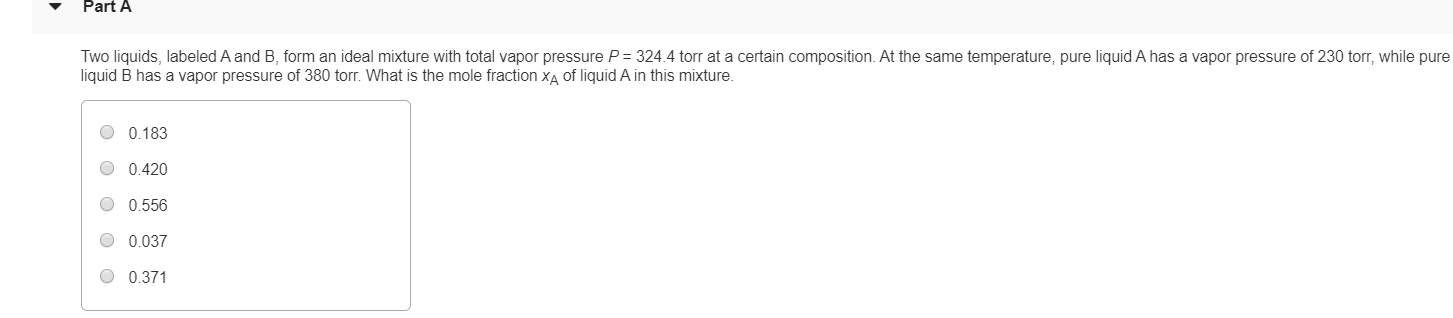Part A Two liquids, labeled A and B, form an ideal mixture with total vapor pressure P = 324.4 torr at a certain composition. At the same temperature, pure liquid A has a vapor pressure of 230 torr, while pure liquid B has a vapor pressure of 380 torr. What is the mole fraction XA of liquid A in this mixture. O 0.183 O 0.420 0.556 O 0.037 0.371

• ### At 300 K, the vapor pressure of pure liquid A and B is 200 mmHg and...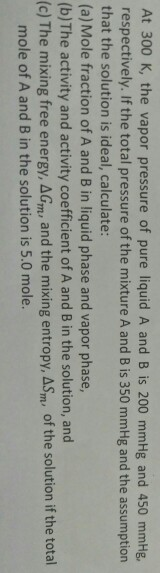At 300 K, the vapor pressure of pure liquid A and B is 200 mmHg and 450 mmHg, respectively. If the total pressure of the mixture A and B is 350 mmHg and the assumption that the solution is ideal, calculate: (a) Mole fraction of A and B in liquid phase and vapor phase, (b) The activity and activity coefficient of A and B in the solution, and (c) The mixing free energy, Agm, and the mixing entropy, Asm, of...

• ### 4. At 300 K, the vapor pressure of pure liquid A and B is 200 mmHg...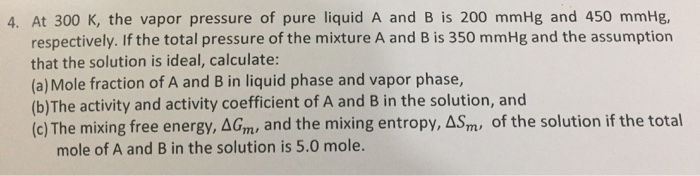4. At 300 K, the vapor pressure of pure liquid A and B is 200 mmHg and 450 mmHg, respectively. If the total pressure of the mixture A and B is 350 mmHg and the assumption that the solution is ideal, calculate: (a) Mole fraction of A and B in liquid phase and vapor phase, (b) The activity and activity coefficient of A and B in the solution, and (c) The mixing free energy, Agm, and the mixing entropy, Asm,...